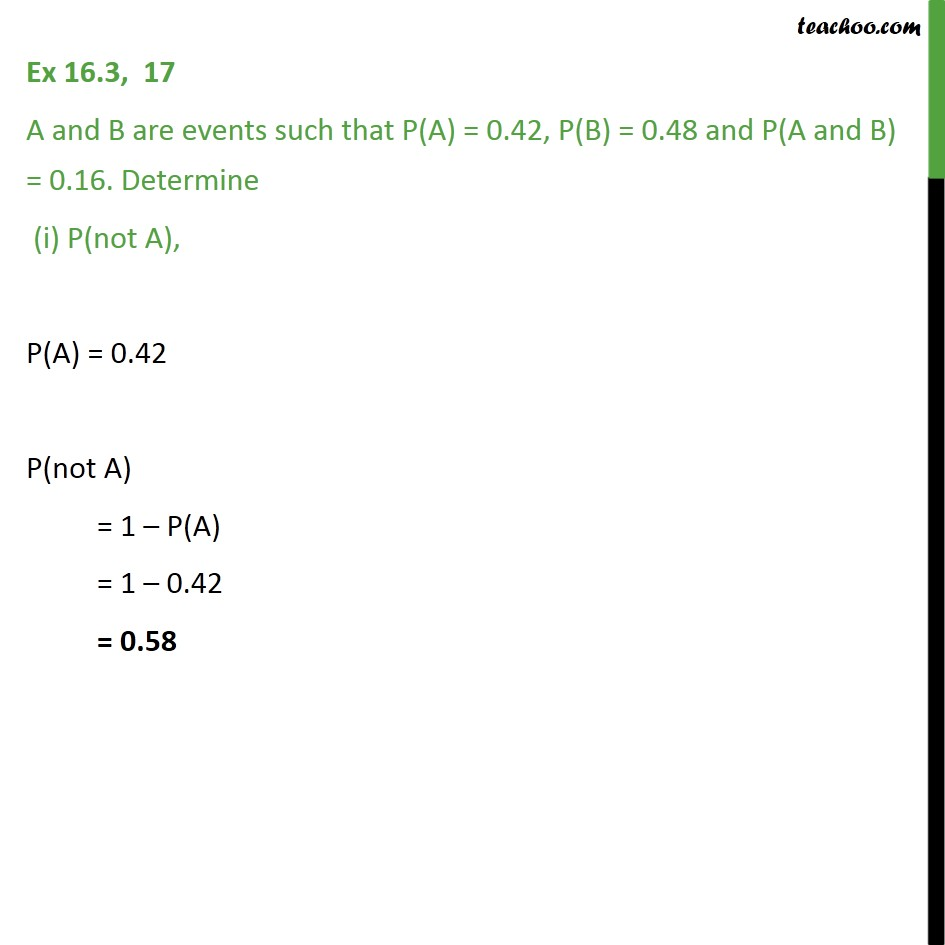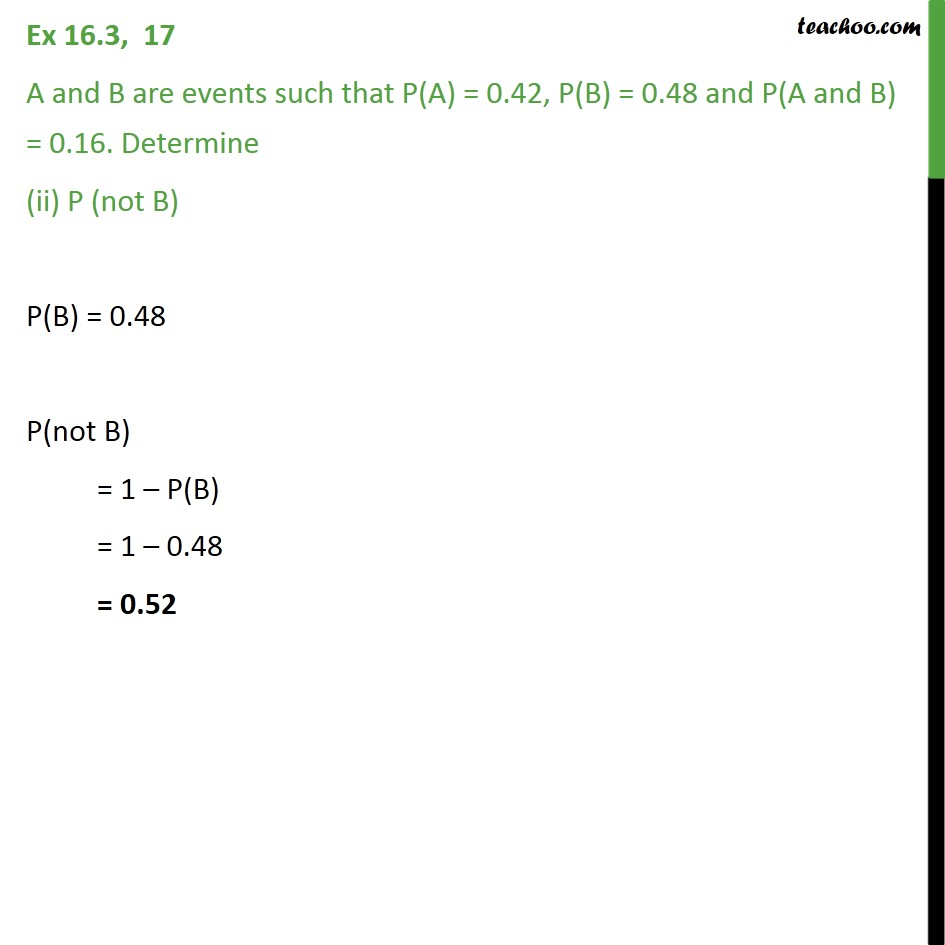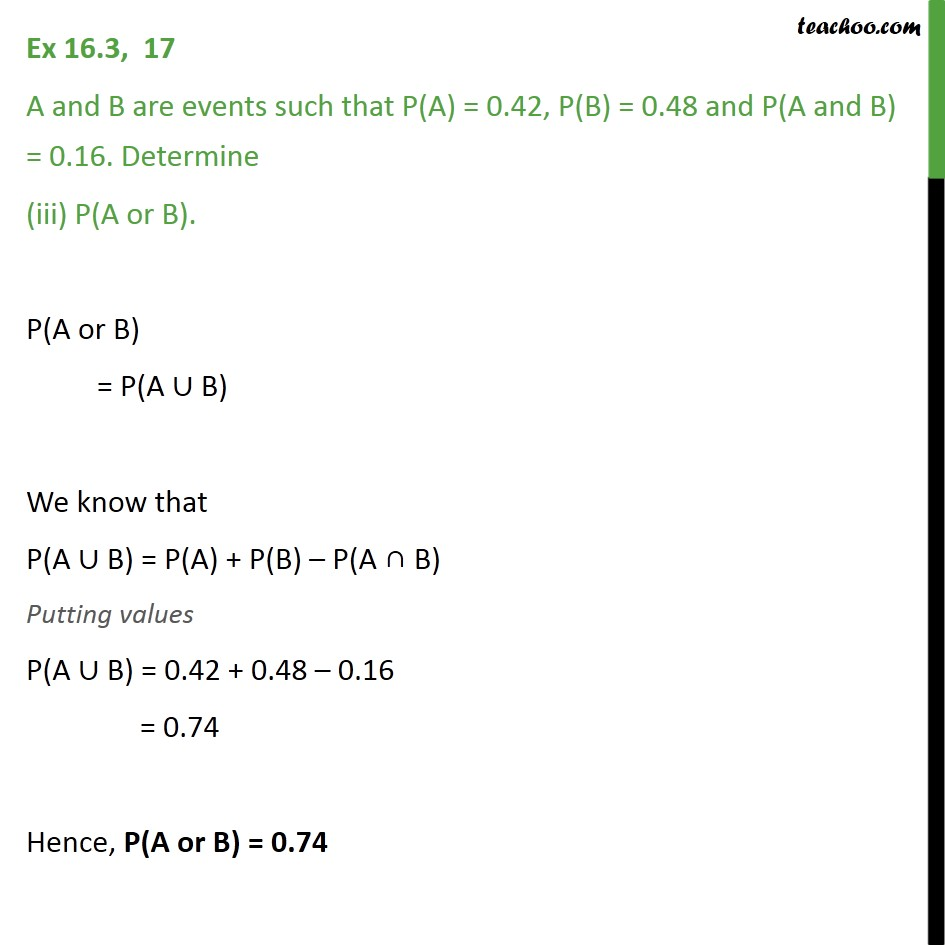Ex 14.2

Chapter 14 Class 11 Probability
Serial order wiseLearn in your speed, with individual attention - Teachoo Maths 1-on-1 Class

### Transcript

Ex 14.2, 17 A and B are events such that P(A) = 0.42, P(B) = 0.48 and P(A and B) = 0.16. Determine (i) P(not A), P(A) = 0.42 P(not A) = 1 P(A) = 1 0.42 = 0.58 Ex 14.2, 17 A and B are events such that P(A) = 0.42, P(B) = 0.48 and P(A and B) = 0.16. Determine (ii) P (not B) P(B) = 0.48 P(not B) = 1 P(B) = 1 0.48 = 0.52 Ex 14.2, 17 A and B are events such that P(A) = 0.42, P(B) = 0.48 and P(A and B) = 0.16. Determine (iii) P(A or B). P(A or B) = P(A B) We know that P(A B) = P(A) + P(B) P(A B) Putting values P(A B) = 0.42 + 0.48 0.16 = 0.74 Hence, P(A or B) = 0.74